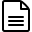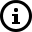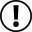# Formula

The Formula feature enables users to modify the visualization of data in specific widgets. Located inside the configuration tab of most widgets, the Formula can be applied to all fields of a stored register, including variable, value, unit, series, location, and metadata.The system operates by receiving input data from a variable in your bucket, applying a formula to generate a temporary output value, which is then displayed in the widget. It's important to note that this output is not saved as a variable in the bucket.In summary, there's two types of formula you can use, the Simple and the Dynamic Formulas.Notice that by default Formula doesn't modify any data in your buckets, it only modifies the visualization. However, you can modify your variable data before its stored in your device's buckets by utilizing the "Allow Edit" option available in the "Edit" tab of certain widgets. This can be achieved by using either the Simple or Dynamic Formulas. To identify the widgets that offer this functionality, refer to the list of widgets available at TagoIO. Be aware that this behavior only affects new data inputs or edits made from TagoRUN. Widgets in the Admin Dashboard won't apply this feature.

# Simple Formulas

This particular formula type is designed to modify the input data you select as a variable within your widget. You have the option to choose between two different source types for this formula: Fixed or Variable.

## Source of the formula

You can choose to enter your formula directly into the Fixed field or select a variable to contain the formula in the JSON field labeled 'value'. If you opt to use the Fixed field, simply enter the formula directly into the widget.

When selecting a Variable as the source for your formula, make sure to select the appropriate variable and ensure that the formula is stored in its 'value' field. Additionally, you can add a 'unit' to the variable, which can be used to display the value later. For instance, if you have a variable that converts temperature from Fahrenheit to Celsius, it might look something like this:
```{
"variable": "equation_f_c",
"unit"    : "°C",
"value"   : "(\$value\$-32)*5/9"
}```There are several methods to add a variable that contains a formula. For example, you can use the Input Form WidgetDevice EmulatorInput Control Widget, or any other tool that enables you to input data to your bucket.

## Operators

These are some of the operators available for you to use:
 Operators Name Example +, - add, subtract \$value\$ + 1000 /, * divide, multiply \$value\$ / 2.14 to, in unit conversion \$value\$ inch to cm ? : conditional expression \$value\$==1 ? \$VALUE\$ ON : OFF >>, << arithmetic shifts \$VALUE\$ >> 1 sqrt, sin, log math operations sqrt (\$value\$)

More options for Operators can be found on Math.jsBecause not all options are available for the Formula, make sure you test it before deploying your solution.

You can also use input fields other than \$value\$; for example:

 JSON Field Example \$variable\$ '\$variable\$' \$unit\$ equalText('\$UNIT\$', 'C') ? \$VALUE\$  degC to degF : \$VALUE\$ \$serie\$ \$serie\$ \$location.lat\$ \$location.lat\$ \$location.lng\$ concat('\$location.lat\$',';','\$location.lng\$') \$metadata.\$ '\$metadata.color\$' all JSON fields \$*\$

## Formula application

Let's say that you want to visualize a 'temperature' in Fahrenheit, but your device stored it in Celsius, you can use one of these formulas:
``` \$VALUE\$ celsius in fahrenheit
```
` \$VALUE\$ degC to degF  `
` (\$VALUE\$ × 9/5) + 32`
More examples are available in the dropdown list in the Formula field.
You can see more options by accessing the Evaluate session of the Math.js documentation that we used as a reference.

## Units for the output variables

In the example above, you will want to see the output value showing the unit °F, instead of the original °C because you converted the value to a new scale.
You have options to define the origin of the unit to be displayed using the field Unit Origin. Learn the options to

## Minimum and Maximum limits

The formula will not adjust the limits of the widgets. The Min and Max values of the range are still solely controlled by the settings defined in the 'Data Range' tab.

# Dynamic Formulas

Dynamic Formulas work differently than its simpler counterpart as it enables you to use formulas in conjunction with your end-users preferences. This means that your users can decide what best suits their needs and the application will automatically adjust itself to the user's configured preference.

For example, you can provide your end-users with the ability to choose between Celsius, Fahrenheit, or Kelvin as their preferred temperature unit in their Account Settings.To utilize the Dynamic Formulas feature, you must first create a new visualization preference in TagoRUN using the Custom Settings feature. You'll later use these settings in the configuration tab to apply the formulas.

The Dynamic Formula configuration tab has the following options:1. Source: The source of the preference you want to apply to your formula, being either your user's preferences or user's browser preferences.
2. Option: Any custom visualization you create in the Custom Settings will be available here.
3. Condition: Defines a value or an option from your Custom Settings to be checked before applying the formula.
4. Formula Type: Defines if you will use a Fixed or a Variable formula type.
5. Formula: The formula you want to apply.

• # Related Articles

• ## Displaying Units

You can setup your widget to present the unit of a variable (e.g. °C, °F, km, mm) in different ways. Under the tab 'Formula', select the option for the origin of the unit that better works for you. 1. Unit from the original variable This option will ...
• ## Gauge - Data Range & Format

Data Range & Format is the place where you can define the format and also the range of the data inside the gauge. Range Limits In the Range limits section you can set the minimum and maximum value displayed in the gauge. There are two different types ...
• ## Dynamic Table Widget

Dynamic tables are tables that dynamically populate as your data arrive while keeping the history of the previous data in each row. Editing the dynamic table It is possible to customize widget color, apply a formula to columns, show time column, show ...
• ## Static Table Widget

Static tables are tables where each cell represents a different piece of information, and it can either be fixed text or data from a variable. Editing the static table It is possible to customize widget color, apply a formula to a cell, edit options, ...
• ## Arduino

This is a quick example using Arduino Zero to send data to Tago. For the connectivity board, we selected the shield Atmel WiFi101. Learn about the Arduino Zero and how to get started. In this example, let’s send the temperature reading from the ...